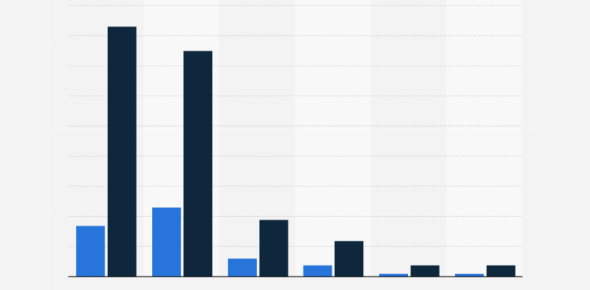# Statistics Questions: MCQ Quiz!

15 Questions | Total Attempts: 105Settings.

• 1.
Find the mean in the following numbers: 9, 8, 15, 8, 20.
• A.

12

• B.

16

• C.

15

• D.

21

• 2.
[Blank]   is the median of the set of data. 15,17, 25, 28, 30.
• 3.
In distribution the if the value of the variate is repeated, it is called median.
• A.

True

• B.

False

• 4.
The ___________ is the middle value when the data are in numerical order, or the mean of the two middle values if there are an even number of items.
• A.

Mode

• B.

Median

• C.

Range

• D.

A.M

• 5.
The ___________ is the difference between the least and greatest values in the set of numbers.
• A.

Mode

• B.

Median

• C.

Frequency

• D.

Range

• 6.
Mode =
• A.

L+(h(f-f1))/(2f - f1 - f2)

• B.

L+(h(f-f1))/(2f - f)

• C.

L+(h(f-f1))/( f- f2)

• D.

L+((f-f1))/(2f - f- f2)

• 7.
Find the mode  of  the following data 6,8,2,5,9,5,6,5,2,3.
• A.

2

• B.

9

• C.

6

• D.

5

• 8.
The ________ is the value or values that occur most often.
• A.

Median

• B.

Range

• C.

Mode

• D.

Frequency

• 9.
The ____________ is the sum of the items divided by the number of items in a set.
• A.

Mode

• B.

Mean

• C.

Median

• D.

Frequency

• 10.
Find the range of the set of data. 100, 43, 26,100,15.
• A.

26

• B.

85

• C.

100

• D.

43

• 11.
Over 5 days, Jason jogged 6.5 miles, 5 miles, 2 miles, 2 miles, and 4.5 miles. Find the mean distance that Jason jogged?
• A.

3.2

• B.

4.6

• C.

3

• D.

4

• 12.
If the mean is  24.6  and  mode  is  26.1. Find  the  median.
• A.

24

• B.

23

• C.

27

• D.

25.1

• 13.
If there is only one mode then it is called?
• A.

Mode

• B.

Multimodal

• C.

Unimodal

• D.

Bimodal

• 14.
Median is the --------  Quartile.
• A.

Fourth

• B.

Second

• C.

Third

• D.

First

• 15.
Mode  =  3 median -   ------------
• A.

3 mean

• B.

4 mean

• C.

5 mean

• D.

2 mean

Related TopicsBack to top